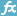(Example) Create a Formula to Calculate the Total Hours Requested for the Create Absence Request eForm

To design the Total Hours Requested formula form control for the Create Absence Request eForm, do the procedure in this topic.

Procedure

1. On the Form Control Quick Menu, click Formula.
2. The Formula Builder screen shows.
3. In the Formula field, enter this formula.
sum( \${LeaveDetails/Hours:[*]})
4. To make sure the formula is correct, click Validate.
5. Click OK.
6. The eForm Builder shows the Total Hours Requested formula field for the Create Absence Request eForm.